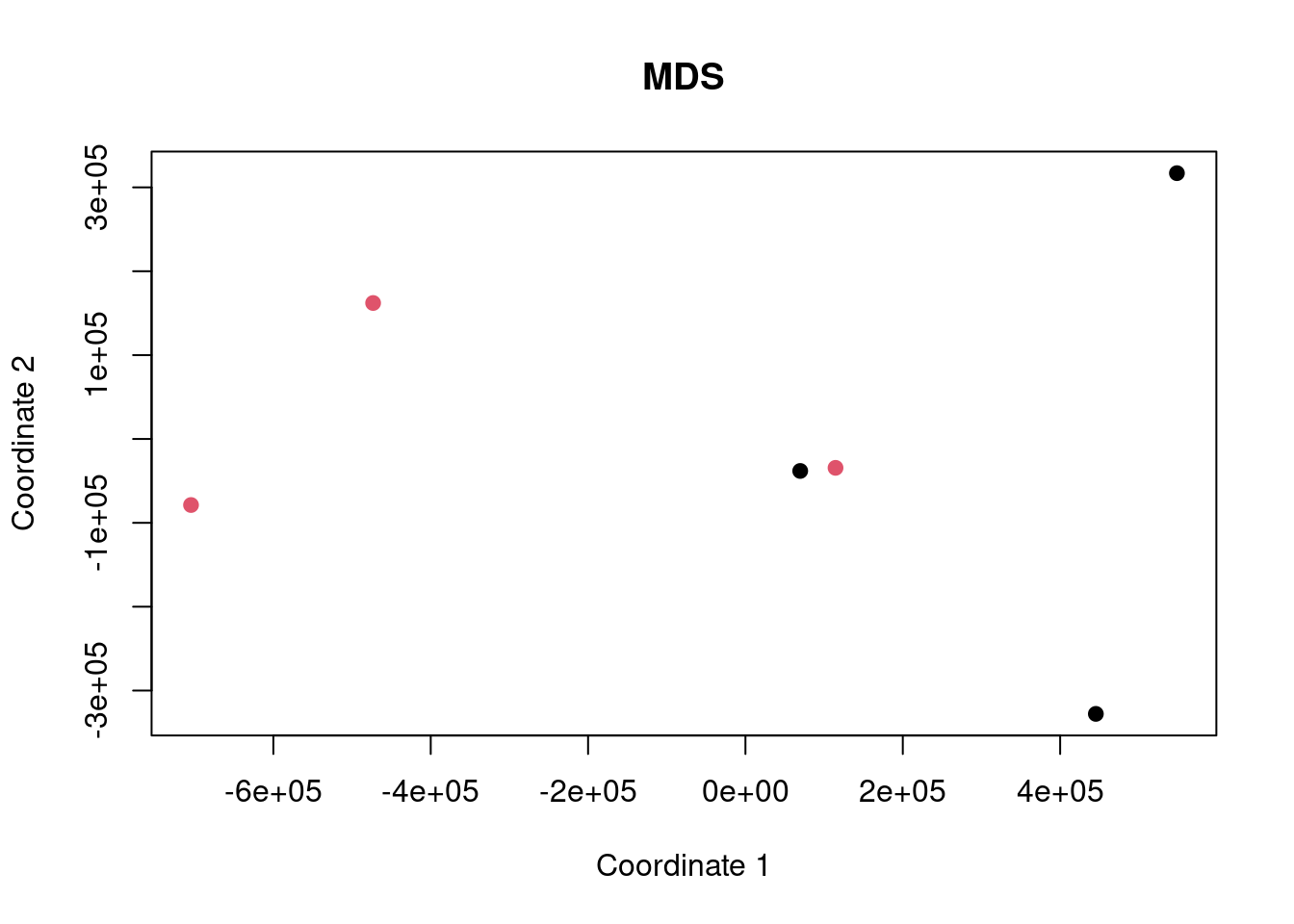## Intro

Here we are performing an analysis of some gene expression data to demonstrate the difference between ORA and FCS methods and to highlight the differences caused by improper background gene set use.

The dataset being used is SRP247621 and we are comparing the fibroblast cells from LHON patients (case) versus healthy controls.

Data are obtained from http://dee2.io/

``````suppressPackageStartupMessages({
library("getDEE2")
library("DESeq2")
library("clusterProfiler")
library("mitch")
library("kableExtra")
library("eulerr")
})``````

## Get expression data

I’m using some RNA-seq data looking at the difference in fibroblast gene expression between control and LHON patients.

``````name="SRP247621"
samplesheet <- mdat[grep("SRP247621",mdat\$SRP_accession),]
samplesheet<-samplesheet[order(samplesheet\$SRR_accession),]
samplesheet\$trt<-as.factor(c(1,1,1,2,2,2,0,0,0)) # exclude carriers for simplicity
samplesheet <- samplesheet[which(samplesheet\$trt!=2),]
s1 <- samplesheet

s1 %>% kbl(caption = "sample sheet") %>% kable_paper("hover", full_width = F)``````
sample sheet
SRR_accession QC_summary SRX_accession SRS_accession SRP_accession Sample_name GEO_series Library_name trt
204421 SRR11040359 PASS SRX7692166 SRS6118270 SRP247621 GSM4300731 GSE144914 1
204422 SRR11040360 WARN(3,4,6) SRX7692167 SRS6118272 SRP247621 GSM4300732 GSE144914 1
204423 SRR11040361 PASS SRX7692168 SRS6118271 SRP247621 GSM4300733 GSE144914 1
204427 SRR11040365 PASS SRX7692172 SRS6118276 SRP247621 GSM4300737 GSE144914 0
204428 SRR11040366 PASS SRX7692173 SRS6118277 SRP247621 GSM4300738 GSE144914 0
204429 SRR11040367 PASS SRX7692174 SRS6118278 SRP247621 GSM4300739 GSE144914 0
``w<-getDEE2("hsapiens",samplesheet\$SRR_accession,metadata=mdat,legacy = TRUE)``
``````## For more information about DEE2 QC metrics, visit
##     https://github.com/markziemann/dee2/blob/master/qc/qc_metrics.md``````
``````x<-Tx2Gene(w)
x<-x\$Tx2Gene

# save the genetable for later
gt<-w\$GeneInfo[,1,drop=FALSE]
gt\$accession<-rownames(gt)

# counts
x1<-x[,which(colnames(x) %in% samplesheet\$SRR_accession)]``````

Here show the number of genes in the annotation set, and those detected above the detection threshold.

``````# filter out lowly expressed genes
x1<-x1[which(rowSums(x1)/ncol(x1)>=(10)),]
nrow(x)``````
``##  39297``
``nrow(x1)``
``##  14288``

Now multidimensional scaling (MDS) plot to show the correlation between the datasets. If the control and case datasets are clustered separately, then it is likely that there will be many differentially expressed genes with FDR<0.05.

``plot(cmdscale(dist(t(x1))), xlab="Coordinate 1", ylab="Coordinate 2", pch=19, col=s1\$trt, main="MDS")``## Differential expression

Now run DESeq2 for control vs case.

``y <- DESeqDataSetFromMatrix(countData = round(x1), colData = s1, design = ~ trt)``
``## converting counts to integer mode``
``## factor levels were dropped which had no samples``
``y <- DESeq(y)``
``## estimating size factors``
``## estimating dispersions``
``## gene-wise dispersion estimates``
``## mean-dispersion relationship``
``## final dispersion estimates``
``## fitting model and testing``
``````de <- results(y)
de<-as.data.frame(de[order(de\$pvalue),])
rownames(de)<-sapply(strsplit(rownames(de),"\\."),"[[",1)
head(de) %>% kbl() %>% kable_paper("hover", full_width = F)``````
baseMean log2FoldChange lfcSE stat pvalue padj
ENSG00000233948 156.47249 -2.955957 0.3146608 -9.394106 0 0e+00
ENSG00000103326 241.05789 1.543670 0.2180326 7.079999 0 0e+00
ENSG00000268861 72.30512 -6.854266 1.0078846 -6.800645 0 0e+00
ENSG00000175221 419.46036 1.246384 0.1836166 6.787967 0 0e+00
ENSG00000273542 17.71562 21.242888 3.1581883 6.726289 0 0e+00
ENSG00000168140 1121.18373 1.113196 0.1771604 6.283553 0 7e-07

Now let’s have a look at some of the charts showing differential expression. In particular, an MA plot and volcano plot.

``````maplot <- function(de,contrast_name) {
sig <-subset(de, padj < 0.05 )
up <-rownames(subset(de, padj < 0.05 & log2FoldChange > 0))
dn <-rownames(subset(de, padj < 0.05 & log2FoldChange < 0))
GENESUP <- length(up)
GENESDN <- length(dn)
DET=nrow(de)
SUBHEADER = paste(GENESUP, "up, ", GENESDN, "down", DET, "detected")
ns <-subset(de, padj > 0.05 )
plot(log2(de\$baseMean),de\$log2FoldChange,
xlab="log2 basemean", ylab="log2 foldchange",
pch=19, cex=0.5, col="dark gray",
main=contrast_name, cex.main=0.7)
points(log2(sig\$baseMean),sig\$log2FoldChange,
pch=19, cex=0.5, col="red")
}

make_volcano <- function(de,name) {